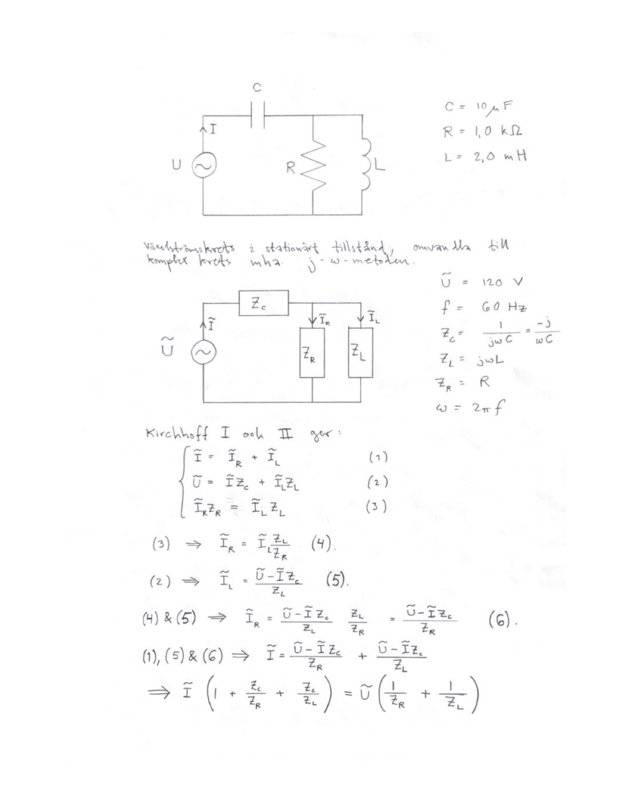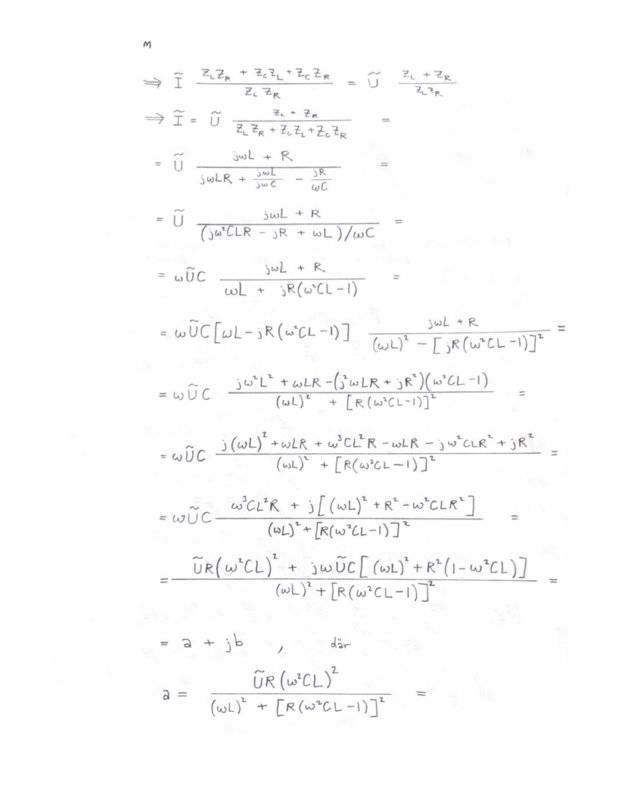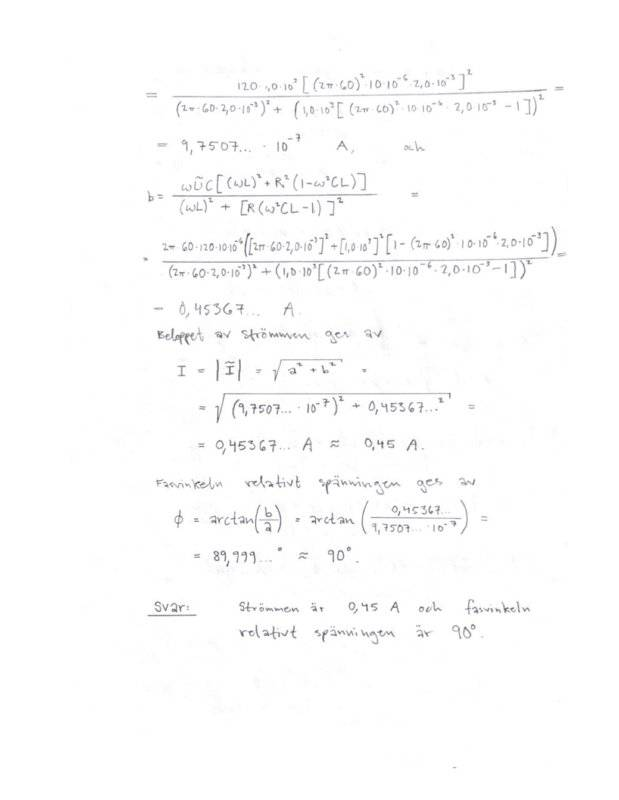# 𝑗 - 𝜔 method alternating current circuit help

magnuol
Homework Statement:
I'm supposed to calculate the current and the phase angle.
Relevant Equations:
Can someone please tell me if this is the correct solution?
I'm supposed to calculate the current and the phase angle. Can someone please tell me if this is the correct solution?Homework Helper
Gold Member
I got the same answer as you. I did not follow every step of your work. While the angle does round to 90 degree, I think it's important to know that it can never equal 90, and will be slightly less.

magnuol
I got the same answer as you. I did not follow every step of your work. While the angle does round to 90 degree, I think it's important to know that it can never equal 90, and will be slightly less.
You got the same answer regarding the value of the current as well?

Mentor
Your current magnitude and angle are both correct.

magnuol
Your current magnitude and angle are both correct.
Does it seem accurate that the order of the real component (a) is 10^(-7) when the imaginary component (b) is that much bigger?

Mentor
Yes. That's why the phase angle is so close to 90°.

Homework Helper
Gold Member
You got the same answer regarding the value of the current as well?
Yes, I got the same magnitude and angle for current I

Homework Helper
Gold Member
Yes, I got the same magnitude and angle for current I
For whatever it's worth, I got the same real and imaginary parts for the current as @magnuol .

•